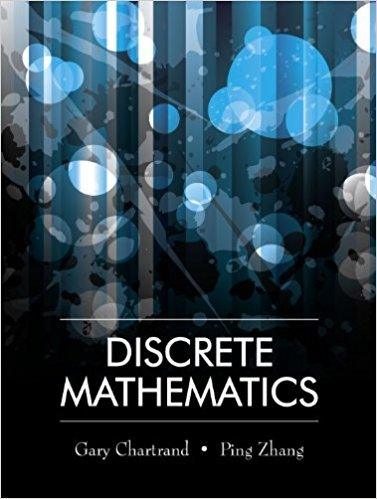×
×

# Disprove the following by providing a counterexample in each case. (a) If a and b are ofISBN: 9781577667308 385

## Solution for problem 11 Chapter 3

Discrete Mathematics | 1st Edition

• Textbook Solutions
• 2901 Step-by-step solutions solved by professors and subject experts
• Get 24/7 help from StudySoup virtual teaching assistantsDiscrete Mathematics | 1st Edition

4 5 1 308 Reviews
15
2
Problem 11

Disprove the following by providing a counterexample in each case. (a) If a and b are of opposite parity, then 2a + 3b is odd. (b) If x is a real number, then (x + 1)2 > 0.

Step-by-Step Solution:
Step 1 of 3

L9 - 10 An important property of continuous functions is the Intermediate Value Theorem (IVT): Suppose f is continuous on [a,b]ad M is any number between f(a)and f(b), then y=f(x) Corollary....

Step 2 of 3

Step 3 of 3

##### ISBN: 9781577667308

Unlock Textbook Solution